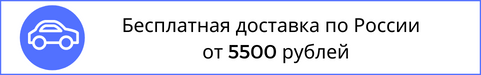Время работы (по Москве)
пн • вт • ср • чт • пт • сб • вс
9-20
Звонок по России бесплатный# Mathematics. Textbook

Артикул NF0018543
ISBN 978-5-9704-5689-7 ГЭОТАР-Медиа Павлушков И.В., Розовский Л.В., Наркевич И.А. 2021 320 твердый

1040 руб.

В наличии

The textbook corresponds to the curriculum of the Mathematics discipline in pharmaceutical and medical higher educational institutions, based on the Federal State Educational Standard for Higher Professional Education of the Third Generation.

The textbook presents a course of mathematics that includes basic elementary functions, diff erential calculus of functions of one variable, elements of diff erential calculus of multiple variable functions, integral calculus of one variable functions, diff erential equations of the fi rst and second order, fundamentals of probability theory and mathematical statistics.

In the textbook, there are detailed explanations of theoretical material and a large number of examples and issues fully explained.

It is intended for undergraduate and postgraduate students of medical and pharmaceutical universities, doctors, pharmacists and individuals, who study mathematics and mathematical statistics by themselves.

Отзывов пока нет.